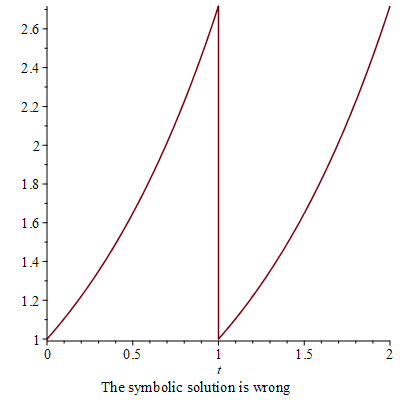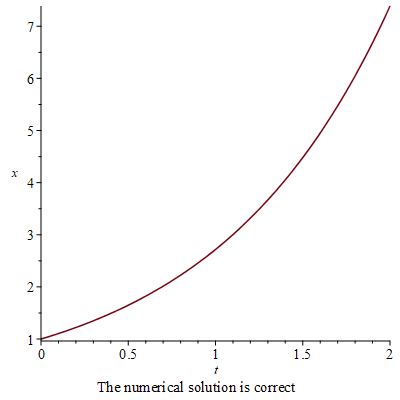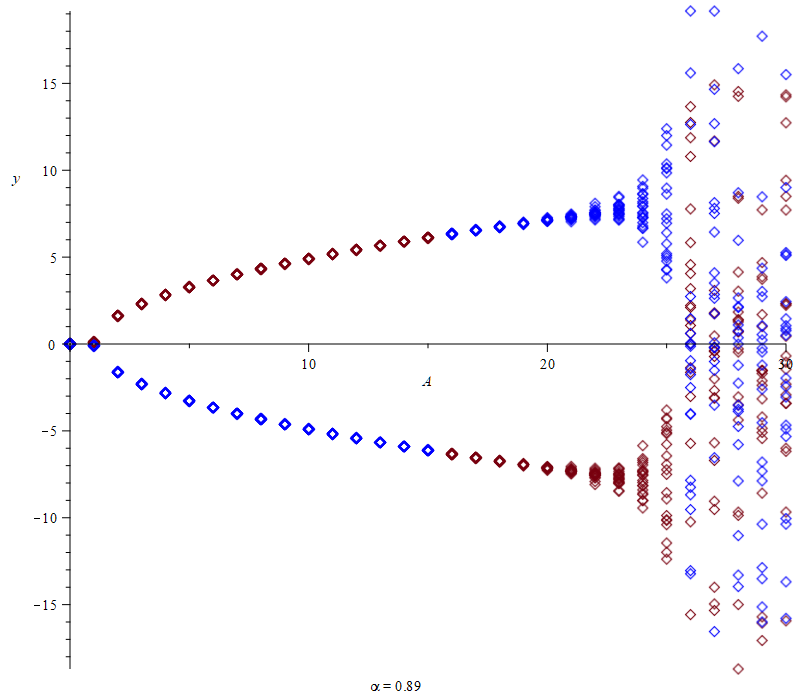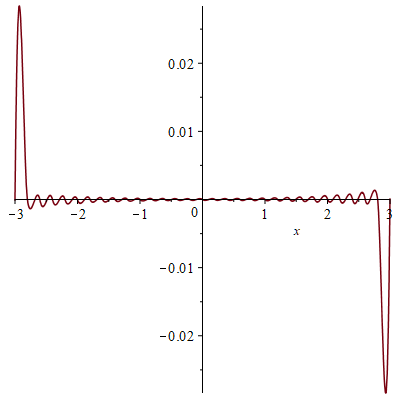## 11289 Reputation

15 years, 54 days

## Yet another...

Yet another Dane with a corrupt file. Something is rotten in the state of Denmark.

## Thanks...

@vv I just tried the conversion from 2D to 1D using lprint. It works. Thank you.

## A bug clearly...

@AmirHosein Sadeghimanesh This is clearly a bug. Since I use 1D-input (aka Maple notation) , where double inequalities like 0 < x < 1 are not allowed I had to rewrite your input. I got the same results as you do though.
I tried without abs and got 29/360 with both piecewise and Heaviside.
I tried replacing abs(F) with sqrt(F^2) just in case, but (as they ought in this case) they gave the same result (although wrong).

```restart;
q1:=-k5*x1*x2+k1*x1;
q2:=-k5*x1*x2+k2*x2;
p1:=piecewise(q1<=0,0,q1>=1,0,1);
p2:=piecewise(q2<=0,0,q2>=1,0,1);
F:=(k5*x2-k1)*(k5*x1-k2)-k5^2*x1*x2;
int(F*p1*p2,[k1 = 0 .. 1, k2 = 0 .. 1, k5 = 0 .. 1, x1 = 0 .. 1, x2 = 0 .. 1]); # 29/360
int(sqrt(F^2)*p1*p2,[k1 = 0 .. 1, k2 = 0 .. 1, k5 = 0 .. 1, x1 = 0 .. 1, x2 = 0 .. 1]); # infinity
int(abs(F)*p1*p2,[k1 = 0 .. 1, k2 = 0 .. 1, k5 = 0 .. 1, x1 = 0 .. 1, x2 = 0 .. 1]); # infinity
g:=convert(p1*p2,Heaviside);
int(F*g,[k1 = 0 .. 1, k2 = 0 .. 1, k5 = 0 .. 1, x1 = 0 .. 1, x2 = 0 .. 1]); # 29/360
int(sqrt(F^2)*g,[k1 = 0 .. 1, k2 = 0 .. 1, k5 = 0 .. 1, x1 = 0 .. 1, x2 = 0 .. 1]); # -29/360
int(abs(F)*g,[k1 = 0 .. 1, k2 = 0 .. 1, k5 = 0 .. 1, x1 = 0 .. 1, x2 = 0 .. 1]); #  -29/360
```

## Title invisible in notification from Map...

@Mariusz Iwaniuk It would be nice if your title in comments contained more than just a period. In the notifications from MaplePrimes the link to those comments are basically invisible. Until now I have thought there were none.

## Bug in convert/Heaviside...

@Mariusz Iwaniuk There is a bug in convert/Heaviside, which affects your worksheet:

```restart;
p:=piecewise(t <> 8*n, y(t), t = 8*n, 0);
pH:=convert(p, Heaviside);```

The output from the conversion is   -y(t)*Dirac(t-8*n)+y(t), which is clearly not the same as p.
Just do:

```int(eval(p,{y(t)= t, n=1}),t=0..10);
int(eval(pH,{y(t)= t, n=1}),t=0..10);
```

Results 50 and 42.

## Bug in convert/Heaviside...

@mmcdara It appears that the bug in dsolve is due to a bug in convert/Heaviside:

```restart;
ode:=diff(x(t),t)=piecewise(t=1,0,x(t));
convert(ode,Heaviside);
```

Result: diff(x(t), t) = -x(t)*Dirac(t-1)+x(t), which is clearly not the same as ode.
This bug is at least as old as Maple 8.

## I don't agree...

@mmcdara Notice that before dsolve sees the ode the right side with `if`:
`if`(t=1, 0, x(t));
evaluates to x(t) for the trivial reason that the name t is not equal to 1.
That is why you need piecewise.

## Explain please + a bug in dsolve/symboli...

@radaar I don't quite understand what you mean.

But incidentally, I found a bug in dsolve/symbolic:

```restart;
ode:=diff(x(t),t)=piecewise(t=1,0,x(t));
sol:=dsolve({ode,x(0)=1});
odetest(sol,ode);
plot(rhs(sol),t=0..2,caption="The symbolic solution is wrong");
res:=dsolve({ode,x(0)=1},numeric);
plots:-odeplot(res,[t,x(t)],0..2,caption="The numerical solution is correct");
```

The symbolic solution is wrong, the numerical one is correct.## Diagram...

@torabi Well, you didn't really answer my question about the amplitude: What does that mean when the attractor is rather complicated and not just a point?
So what I decided to do was to sample the y-values for various time values equidistantly spaced after an initial settling period.
The code follows.

```## This assumes that you have executed the definition of my version of fdsolve given earlier.
## option remember in the procedure means that if you repeat any of this for the same
## A-value then it won't take long.
##That is why I stick to a previously defined list of A-values.
##
F:=omega*x-y^2;
G:=mu*(z-y);
H:=A*y-B*z+x*y;
params:={omega=-2.667,mu=10,B=1}; # A unassigned
## Order alpha, final time T, number of points N. All will be fixed in advance.
alpha:=0.89: T:=20: N:=2000:
solfu:=proc(AA,inits::[numeric,numeric,numeric]) option remember,system; local FGH;
if not AA::numeric then return 'procname(_passed)' end if;
FGH:=unapply~(eval([F,G,H],params union {A=AA}),t,x,y,z);
fdsolve(FGH,alpha,0..T,inits,N)
end proc:
## We shall be using two initial conditions inits1 and inits2:
inits1:=[.1,.1,.1]; inits2:=[-.1,-.1,-.1];
## The A values considered are:
Alist:=[seq(0..30,1)];
sol:=solfu(Alist,inits1); #Test (not wasted because of option remember)
plot(sol[..,[1,3]],labels=[t,y],size=[1200,default]);
## The following animation is not wasted because of option remember.
plots:-animate(plots:-spacecurve,[solfu(A,inits1)[..,2..4] ],A=Alist);
## For each A we pick points beginning with Nb and continuing with a spacing of n points.
## Thus we pick the points numbered Nb+i*n, i=0..floor((N-Nb)/n).
Nb:=N-1000; n:=50;
numpts:=floor((N-Nb)/n)+1; # Number of points chosen for each A
## The distance in time between points will be
n*T/N;
## The procedure Q produces a sequence of points for A given.
Q:=proc(A,inits::[numeric,numeric,numeric]) local sol;
sol:=solfu(A,inits);
seq([A,sol[Nb+i*n,3]],i=0..numpts-1)
end proc;
##
PtList1:=[seq(Q(A,inits1),A=Alist)]: # This is fast because of the animation done earlier.
plot(PtList1,style=point); p1:=%:
PtList2:=[seq(Q(A,inits2),A=Alist)]: # This takes a while.
plot(PtList2,style=point,color=blue); p2:=%:
plots:-display(p1,p2,labels=[A,y],size=[800,700],caption=typeset('alpha'=alpha));
```

The plot:Because of option remember the following won't take long:

```## It shows animations in A of the orbits from inits1 and inits2 in the same coordinate system.
use plots in
a1:=animate(spacecurve,[solfu(A,inits1)[..,2..4] ],A=Alist);
a2:=animate(spacecurve,[solfu(A,inits2)[..,2..4] ],A=Alist);
display(a1,a2)
end use;
```## floatPi...

@Mariusz Iwaniuk A detail about Pi which you have outside evalf and which would cause a problem (easily cured though) in earlier versions of Maple.
In versions from Maple 18 and earlier the option kernelopts(floatPi) didn't exist. The default setting in the Maple releases 2018, 2017, and 2016 is floatPi=true. The option is available in Maple 2015, but is not documented; default is floatPi=false.
What it means having kernelopts(floatPi=true)  is that the presence of a float in a product or sum involving Pi makes Pi evaluate as a float.

```kernelopts(floatPi=true);
Pi*1.0; #Results in a float
Pi+0.0;  #Results in a float
[Pi,1.0]; # Doesn't
```

Personally I don't like that setting and have kernelopts(floatPi=false) in my maple.ini file.
One reason for disliking the setting is that it singles out the constant Pi. It doesn't apply to any other constant.
Example: sqrt(2)*1.0 evaluates to just that, not to a float.

## The error...

@Mariusz Iwaniuk The error is not impressively low:

`plot(sinh(x)-ifunc(5)(x), x = -3 .. 3);`It doesn't appear to improve by using more iterations.
I think you would be better off using finite elements with collocation, i.e. replacing y by a linear combination of basis functions (e.g. monomials) Y with coefficients to be determined. Then evaluate the resulting equation at some points and solve for the unknown coefficients.
In this linear case that approach will be far better.

## Well ......

@torabi Plotting y as a function of A assumes that you have a precise definition of what y is.
You stated earlier that y meant " the amplitude of the y component of the system's attractor".
What does that mean when the attractor is rather complicated and not just a point?

## Dependence on A...

@torabi No I haven't looked much at it.
What I have done is to look at the dependence on A for fixed alpha = 0.89.

Using the version of fdsolve given in the worksheet FracDiff.mw and using the example in the paper, I did the following.

```## Assuming fdsolve defined as in FracDiff.mw
F:=omega*x-y^2;
G:=mu*(z-y);
H:=A*y-B*z+x*y;
paramsA:={omega=-2.667,mu=10,A=AA,B=1}; # A and AA unassigned
params2:=unapply(paramsA, AA);
## Using alpha=0.89:
##
solfu2:=proc(AA) option remember,system; local FGH;
if not AA::numeric then return 'procname(_passed)' end if;
FGH:=unapply~(eval([F,G,H],params2(AA)),t,x,y,z);
fdsolve(FGH,0.89,0..20,[.1,.1,.1],2000)
end proc;
##
sol:=solfu2(27.3); #Test
plot(sol[..,[1,3]],labels=[t,y],size=[1200,default]);
plot(sol[..,[1,3]],y=10..20,labels=[t,y],size=[1200,default]);
plots:-animate(plots:-spacecurve,[solfu2(A)[..,2..4] ],A=[15,20,25,27.3]);
## The next will be fast because solfu2 has option remember:
plots:-animate(plot,[solfu2(A)[..,[1,3]] ,t=10..20],A=[15,20,25,27.3],size=[1200,default]);
```

solfu2(A) just computes the solution for a given value of A.
The plots found are just the orbits of graphs of the solutions.
When t starts at a late value (e.g. as here 10) then perhaps enough time has passed for the orbit to be close to a possible attractor.

## Simplify...

@Seb1123 Stating the obvious (even without having to consult the help):  simplify will do its best to simplify.
Simplification of an equation just means simplifying each side.
In fact you don't need simplify to do that job for your equation. Just write it down and press ENTER:
F*R=(1/2*M*R^2)*(a/R);
The output is F*R = (1/2)*M*R*a.
So you expected simplify to read your mind. To read that you wanted to solve the equation for F?

## Not analytic...

@Federiko My question was basically meant as 'rhetorical'.

If a locally Lebesgue integrable function f defined on [0, infinity[  satisfying for some M >0, some real a, and some t0 >= 0 the inequality
abs(f(t)) <= M*exp(a*t) for a.e. t >=t0  ( "f is exponentially bounded")

then the Laplace transform F(s) exists for all s satisfying Re(s) > a. Furthermore F is analytic in that region. In particular continuous, thus cannot have singularities.
If your F has infinitely many singularities (s[n]) and s[n] -> infinity, then your F cannot be the Laplace transform of an exponentially bounded locally Lebesgue integrable function.

﻿